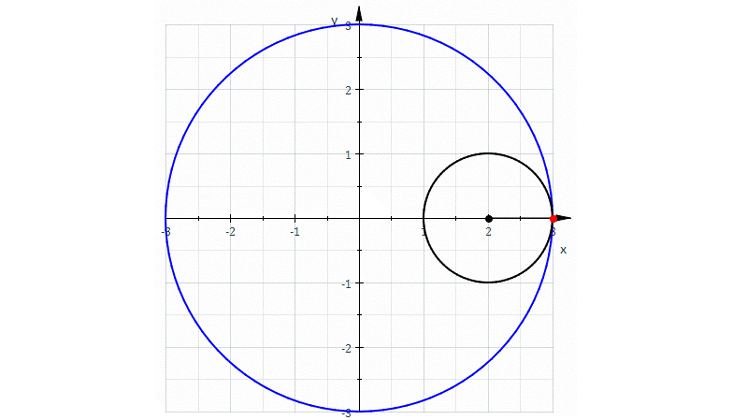## BlogIn my 50s, too old to become a real expert, I have finally fallen in love with algebraic geometry. As the name suggests, this is the study of geometry using algebra. Around 1637, René Descartes laid the groundwork for this subject by taking a plane, mentally drawing a grid on it, as we now do with graph paper, and calling the coordinates x and y. We can write down an equation like x + y = 1, and there will be a curve consisting of points whose coordinates obey this equation. In this example, we get a circle!

It was a revolutionary idea at the time, because it let us systematically convert questions about geometry into questions about equations, which we can solve if we’re good enough at algebra. Some mathematicians spend their whole lives on this majestic subject. But I never really liked it much until recently—now that I’ve connected it to my interest in quantum physics.

If we can figure out how to reduce topology to algebra, it might help us formulate a theory of quantum gravity.